# Inverse Functions

In the previous article, you learned about composite function, in this article, you will learn about inverse functions. The term “inverse” means to “undo” something and which is what the “inverse” of a function do. If a function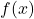find the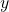value for an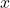value, the inverse of the function does the opposite, meaning it finds thevalue for a givenvalue.

Function and its inverse

Remember from previous articles, that the functionis set of ordered pair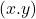, that is, for every inputthere is a one and only.

Suppose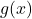is function that takes inputand gives us the value. We are just undoing the function. Therefore, ifis inverse function of, then it is denoted as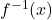.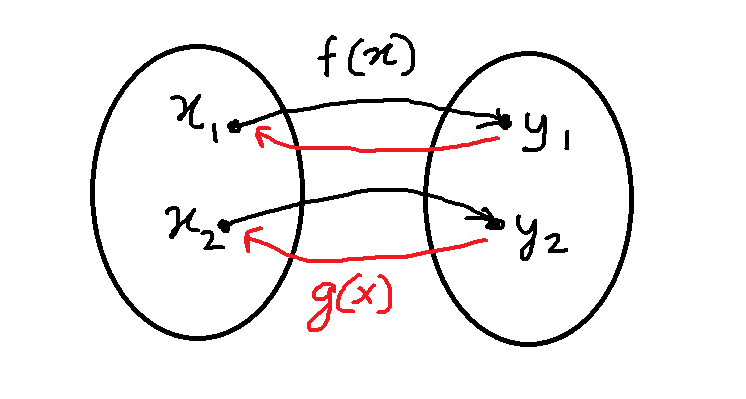Figure 2 – The function g(x) is inverse function with ordered pair (y1, x1), (y2, x2).

The inverse of the functionis the set of ordered pairs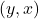.

### One-To-One Relationship

If you look at the figure 2, you will find that there is a one-to-one relationship between functionand its inverse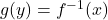.

If there are two functionsand its inverse function, then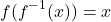Whereis all values in the domain of inverse function. Similarly,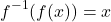Whereis all values in the domain of function.

If a functiondoes not maintain the one-to-one relationship, there is no inverse. Consider the quadratic function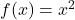. The function has same outputs for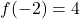and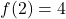.

If we take an inverse of the function which is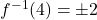implies that there are two values for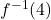. Therefore, inverse is not a function because a function can only have one output for given input.

### Horizontal Line Test

The easiest way to understand whether a function has inverse or not is to perform a horizontal line test on the graph of the function. To understand this concept , we will use our previous example of quadratic function. The graph of function is given below.

We perform a horizontal line test , that is, draw a horizontal line and if the line intersect the graph of functionit has no inverse function. The figure 4 above, shows that the horizontal line intersect the graph of parabola, at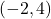and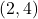. Therefore,has no inverse.

### Graph of Inverse Function

The graph of inverse function, if exists, can be obtained easily by changing the set of ordered pair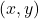to the set of ordered pair.

For example, consider the graph of exponential function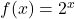which is an exponential function. The function passes the horizontal line test, therefore, an inverse function exists.

The inverse function is set of all ordered pairs. The inverse function of exponential function is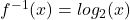.

Note that the inverse function is accepting all positivevalues, and all inverse function (shown in red) will reflect over the line ( in green)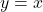. The ordered pairsin exponential function is replaced with ordered pair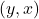.

### How To Find The Inverse Function ?

To find the inverse of the function you must follow the following steps. Ifis a function with an expression, then

1. Writeinstead of.
2. Interchange theandin the equation.
3. Solve the equation forand if the equation does not definein terms of, then there is no inverse. Otherwise, you will have an equation that definesin terms of.
4. Replacewith.

Now, it is necessary to verify the inverse function, that can be done by verifying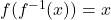and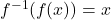. The composition of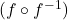and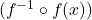is an algebraic proof of inverse function.

Example #1

Find the inverse function of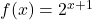.

Solution:

The given equation is. exponential equation and we expect to find a logarithmic function as inverse. But, we will go through all the steps to find the inverse of this function.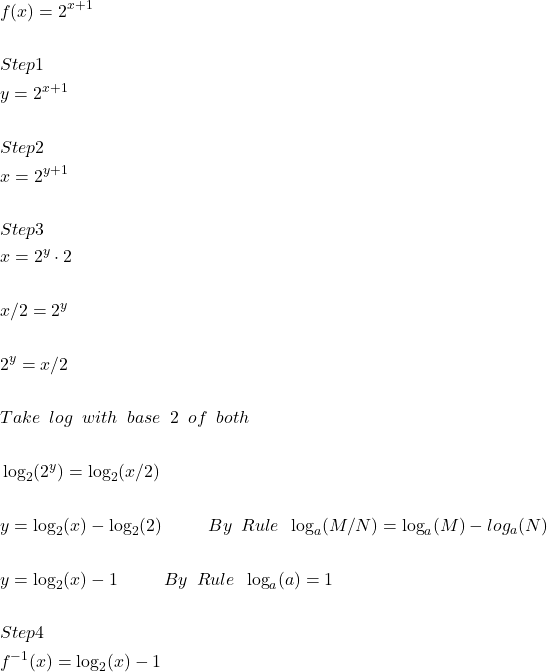Now , we must verify if the inverse function is correct, by using composition of functions. Therefore,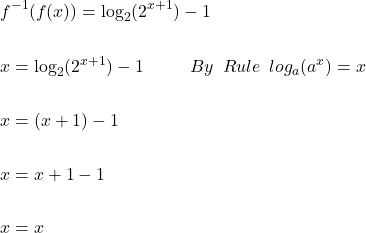Similarly,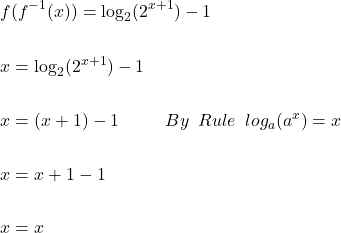Example #2

Find the inverse function of the function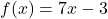.

Solution:

The functionis a linear function. Therefore, an inverse function exists, but we will verify whether inverse exist of not by following our steps to find the inverse of a function.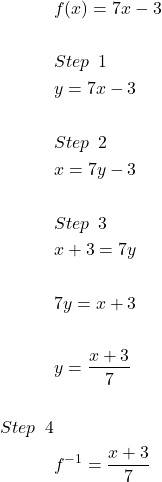Now, we must verify the inverse function.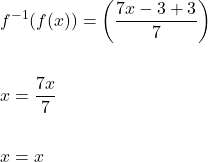Similarly,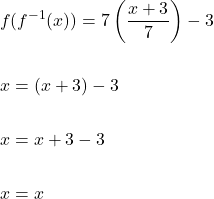### Restricted Domain

It is possible to find an inverse function to functions that does not have any inverse if we restrict the domain. It means we only accept set of ordered pairsof functionfor which there exist ordered pairs.

Consider the graph of absolute value function [latedpage]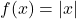. This graph did not pass the horizontal line test and for eachvalue, there exist twovalues.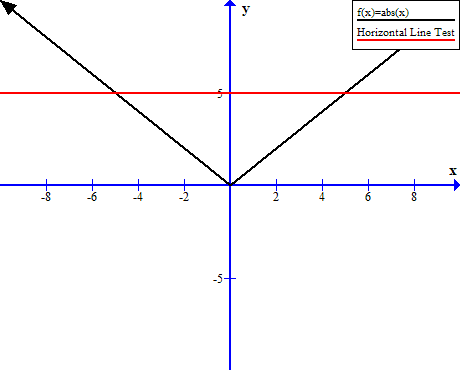Figure 7 – Graph of absolute function is f(x) = |x| and it fails the horizontal line test.

The graph will pass the horizontal line test if we restrict the domain to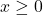, therefore, an inverse of absolute value function exists if we choose values between the interval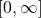.

The inverse of the new restricted absolute function is as follows.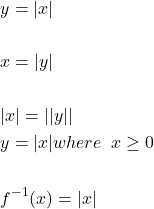We must observe two things,

1. The absolute value cannot be a negative number, therefore,where.
2. The functionand its inverse does not reflect over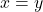, but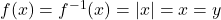.